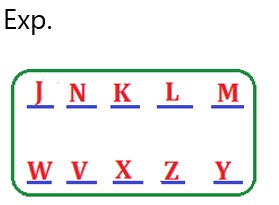# RBI Assistant Pre 2023 Reasoning Ability Quiz –15

## RBI Assistant Pre 2023 Reasoning Ability Quiz

Almost all major competitive exams in the country have a section on Reasoning Ability, and it is also one of the most scoring sections. Candidates can ace this section in exams if they practice regularly in a dedicated manner. So here we are providing you with the RBI Assistant Pre 2023 Reasoning Ability Quiz to help you prepare better. This RBI Assistant Pre 2023 Reasoning Ability Quiz includes all of the most recent pattern-based questions, as well as Previous Year Questions. This RBI Assistant Pre 2023 Reasoning Ability Quiz is totally FREE. Candidates will be provided with a detailed explanation of each question in this RBI Assistant Pre 2023 Reasoning Ability Quiz. Candidates must practice this RBI Assistant Pre 2023 Reasoning Ability Quiz to achieve a good score in the Reasoning Ability Section.

Directions (1-5): Study the given information carefully to answer the given questions:

Ten persons are sitting in two parallel rows containing five persons each, in such a way that there is an equal distance between adjacent persons. In row —1, J, K, L, M, and N are seated (not necessarily in the same order) and all of them are facing south. In row-2, V, W, X, Y and Z are seated (not necessarily in the same order) and all of them are facing north. Therefore in the given seating arrangement each member seated in row faces another member of the other row.

Z sits third to the right of W. V sits second to the left of Z. The person facing V sits to the immediate right of K. Only one person sits between K and M. J is not an immediate neighbour of K. Only two persons sit between J and L. Neither K nor J faces Y.

1. Who amongst the following is facing N?

(a) Y

(b) V

(c) X

(d) W

(e) Z

Ans. b1. Which of the following statements is true regarding M?

(a) M faces one of the immediate neighbours of X.

(b) K is one of the immediate neighbours of M.

(c) None of the given statements is true.

(d) L sits to the immediate right of M.

(e) Only one person sits between M and N.

Ans. d1. Who amongst the following is facing X?

(a) K

(b) L

(c) M

(d) J

(e) N

Ans. a1. What is the position of Z with respect of Y?

(a) Third to the right

(b) second to the right

(c) Immediate left

(d) Immediate right

(e) Second to the left

Ans. c1. Four of the following five are alike in a certain way based on the given arrangement and hence form a group. Which of them does not belong to that group?

(a) M

(b) J

(c) Y

(d) W

(e) N

Ans. eDirections (6-10): In these questions, relationship between different elements is shown in the statements. The statements are followed by conclusions. Study the conclusions based on the given statements and select the appropriate answer. Give answer-

1. Statements: S L I = P E > R; L > Q

Conclusions:       I. P S                II. I > R

(a) If only conclusion II is true

(b) If only conclusion I is true

(c) If both conclusions I and II are true.

(d) If either conclusion I or II is true

(e) If neither conclusion I nor II is true.

Ans. c

Exp.     I. P ≥ S (True)             II. I > R (True)

1. Statements: G > R E = A T S; D A J

Conclusions:       I. T D                II. R > S

(a) If only conclusion II is true

(b) If only conclusion I is true

(c) If both conclusions I and II are true.

(d) If either conclusion I or II is true

(e) If neither conclusion I nor II is true.

Ans. b

Exp.              I. T ≥ D (True)             II. R > S (False)

1. Statements: A B > C D E < F

Conclusions:       I. A E                II. C < F

(a) If only conclusion II is true

(b) If only conclusion I is true

(c) If both conclusions I and II are true.

(d) If either conclusion I or II is true

(e) If neither conclusion I nor II is true.

Ans. a

Exp.             I. A ≥ E (False)             II. C < F (True)

1. Statements: G > R E = A T S; D A J

Conclusions:       I. J > G                II. J = G

(a) If only conclusion II is true

(b) If only conclusion I is true

(c) If both conclusions I and II are true.

(d) If either conclusion I or II is true

(e) If neither conclusion I nor II is true.

Ans. e

Exp.              I. J > G (False)            II. J = G (False)

1. Statements: S L I = P E > R; L > Q

Conclusions:       I. L < R                II. E Q

(a) If only conclusion II is true

(b) If only conclusion I is true

(c) If both conclusions I and II are true.

(d) If either conclusion I or II is true

(e) If neither conclusion I nor II is true.